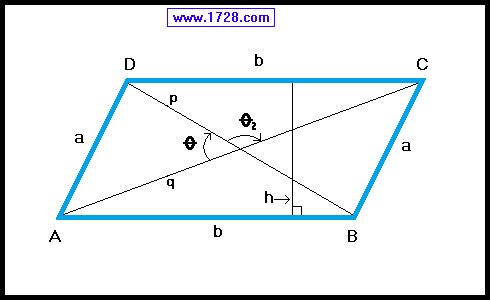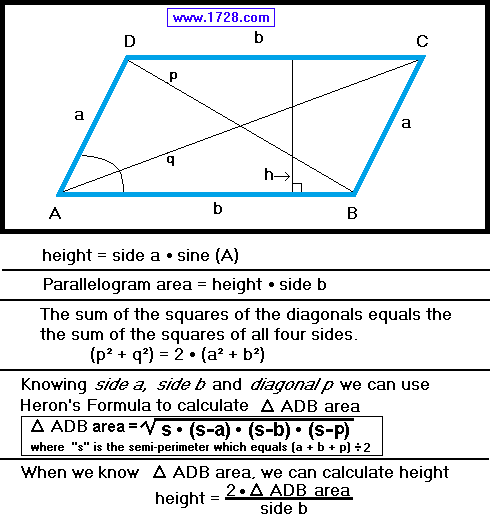Parallelogram Calculator
and Equations
Scroll down for Instructions and Definitions

 Click on the data you know: 2 SIDES and 1 ANGLE 2 DIAGONALS and ANGLE of INTERSECTION 2 SIDES and 'p', the SHORT DIAGONAL BOTH DIAGONALS and Side aFirst, click on one of the choices, enter the data in the appropriate input boxes, then click "CALCULATE".

If an obtuse angle is input, the program will automatically convert it to an acute angle.

Parallelogram Equations & PropertiesParallelogram area = (height × AB) or (height × DC)

Lines AC and DB are called diagonals.
Diagonals bisect each other.
Diagonals are not equal.
Diagonals do not bisect the vertex angles.

Line 'h' is perpendicular to lines AB & DC and is called the height or altitude.

• BOTH pairs of opposite sides are equal.
AB = DC   and   AD = BC

• BOTH pairs of opposite angles are equal.
∠ A = ∠ C   and   ∠ B = ∠ D

∠ A + ∠ D   =   ∠ D + ∠ C =   ∠ C + ∠ B =   ∠ B + ∠ A = 180°

• Each diagonal forms 2 congruent triangles.
Δ ADC = Δ ABC and Δ DAB = Δ DCB

Significant Figures >>>
The default setting is for 5 significant figures but you can change that by inputting another number in the box above.

Answers are displayed in scientific notation and for easier readability, numbers between .001 and 1,000 will be displayed in standard format (with the same number of significant figures.)
The answers should display properly but there are a few browsers that will show no output whatsoever. If so, enter a zero in the box above. This eliminates all formatting but it is better than seeing no output at all.

_____________________